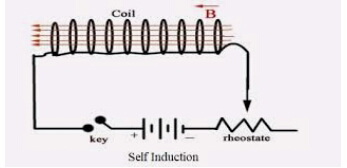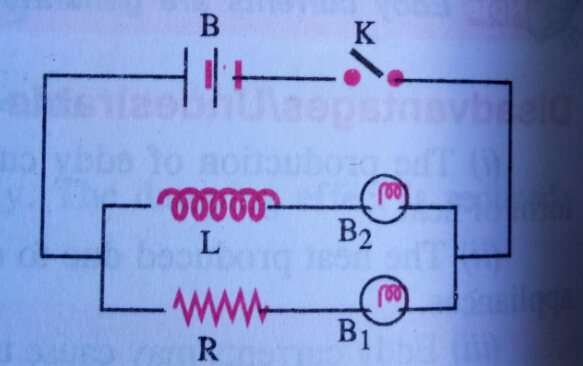# Self induction - Demonstration of self induction effect.

Views

### Self Induction

self induction is not only a single concept in physics but is distributed widely in parts. The applications of this concept are enough to describe it's importance. This is what inspired me to write an article about it. The most interesting thing to know in this article is  "Demonstration of the self induction Effect".

self induction is the property of a coil by virtue of which the coil opposes the growth or decay of the current flowing through it.self induction

#### Let's consider a coil as shown in the figure connected to a battery through a key K.

when the key K is pressed, current in the coil starts increasing. Due to increase of this current in the coil, the magnetic field and the magnetic flux linked with the coil also increases. As a result of this process, induced EMF is set up in the coil.

According to Lenz's Law , the direction of induced EMF is such that it opposes the growth of current in the coil . This delays the current to acquire the maximum value.

When the key K is released, the current in the coil starts decreasing , which results in the decrease of magnetic flux linked with the coil. As a result of this change in magnetic flux, induced EMF is set up in the coil itself.

According to Lenz's Law , the direction of induced EMF is such that it opposes the decay of current in the coil . This delays the current to acquire minimum or zero value.

This property of the coil which opposes the growth or decay of the current in the coil is called self induction.

#### Note:

self induction is also known as inertia of electricity as it opposes the growth or decay of the current in the coil.

#### Demonstration of the self induction Effect:

1) Simple Experiment :

Connect two lamps in parallel to each other, one through the ohmic resistor (R)
and the other one through a coil (L). Connect this combination across a battery (B) through one way key (K).Demonstration of self induction effect.

It is found that the lamp B1  glows immediately, when the key(K) is closed but the lamp  B2 glows slowly.

An  EMF is induced in the coil, when current flows through the coil, which opposes the growth of current in the circuit. Hence glow of lamp B2 is slow.  on the other hand, no induced EMF is produced in the resistor. Hence the lamp B1 receives maximum current as soon as the key (K)  is closed and it glows at once.

when the key K is opened,  lamp B1stops glowing at once but the lamp B2 takes some time to stop glowing. In this case, current begins to decrease through the coil, so again EMF is induced in it. This induced EMF opposes the decay of current in the circuit of lamp B2.

#### 2) Spark is produced in the electric switch when the light is switched off :

The current in the circuit begins to decrease rapidly, when the circuit is switched off.  As a result of this, a large induced EMF is set up across the switch contacts which tries to maintain the current in the circuit. The induced EMF so produced is sufficient to break down the insulation of the air between the switch contacts and hence spark is produced.

#### 3) Non-Inductive coil :

Each coil of resistance box is doubled back on itself while being coiled up. This is done to decrease the self induction. The current in every part of coil is equal and opposite to that of the opposite part of the coil . So the magnetic field around one part is cancelled by the magnetic field around the opposite part. Thus, self induction becomes minimum.

#### Coefficient of self induction or Self Inductance :

Let  I be the current flowing through the coil at any Instant, then the magnetic flux (ɸ) linked with the coil is found to be proportional to the strength of the current (I).

That is;
ɸ ∝ I

or                   ɸ = LI   ................(A)

where L is the constant of proportionality and is known as coefficient of self induction or simply self inductance.

Put I = 1 , we get;

L = ɸ

Thus, coefficient of self inductance of a coil is defined as the magnetic flux linked with the coil, when unit current flows through it.

Also,  according to Faraday's law of electro magnetic induction,  induced EMF  in the coil is given by;

ɛ = − dɸ/dt

Using equation   (A), we get ;

ɛ = − d(LI)/dt

ɛ = − L dI/dt  .................(B)

If rate of decrease of current is unity , that is ;

− dI/dt = 1

then from equation (B) , we get ;

L = ɛ

Thus,  the coefficient of self induction of a coil is defined as the induced EMF produced in the coil through which the rate of decrease of current is unity.

#### Units of Self Induction:

SI unit of self inductance is Henry (H).

Since                                                L = ɛ/dI/dt

∴           1 henry (H)   =  1 volt / 1 ampere second  ̄¹

= 1 V A ̄¹s

Also                                  L = ɸ/I

∴          1 henry (H)  =  1 weber/1 ampere

=  1 wb A ̄¹

i.e.   1 H = 1 VA ̄¹s = 1 wb A ̄¹

#### smaller units of self inductance are :

1 m H = 10 ̄³ H  and 1 μH = 10 ̄⁶ H.

#### Dimensional formula for Self Inductance (L) :

ɛ = L  dI/dt

or            L = ɛ/dI/dt  =  w/dq/dI/dt = w/IdI

or           (L) = ( M¹L²T  ̄²A ̄²)

#### What is an inductor ?

An element of an electric circuit like a tightly wound coil of insulated wire which opposes the change in current flowing through it is called an inductor.

Conclusion:

The article has discussed about the concept of self induction. It provides you the  brief but complete information.

The article discussed the demonstration of the concept of self inductance. More related concepts have been discussed also.

1.